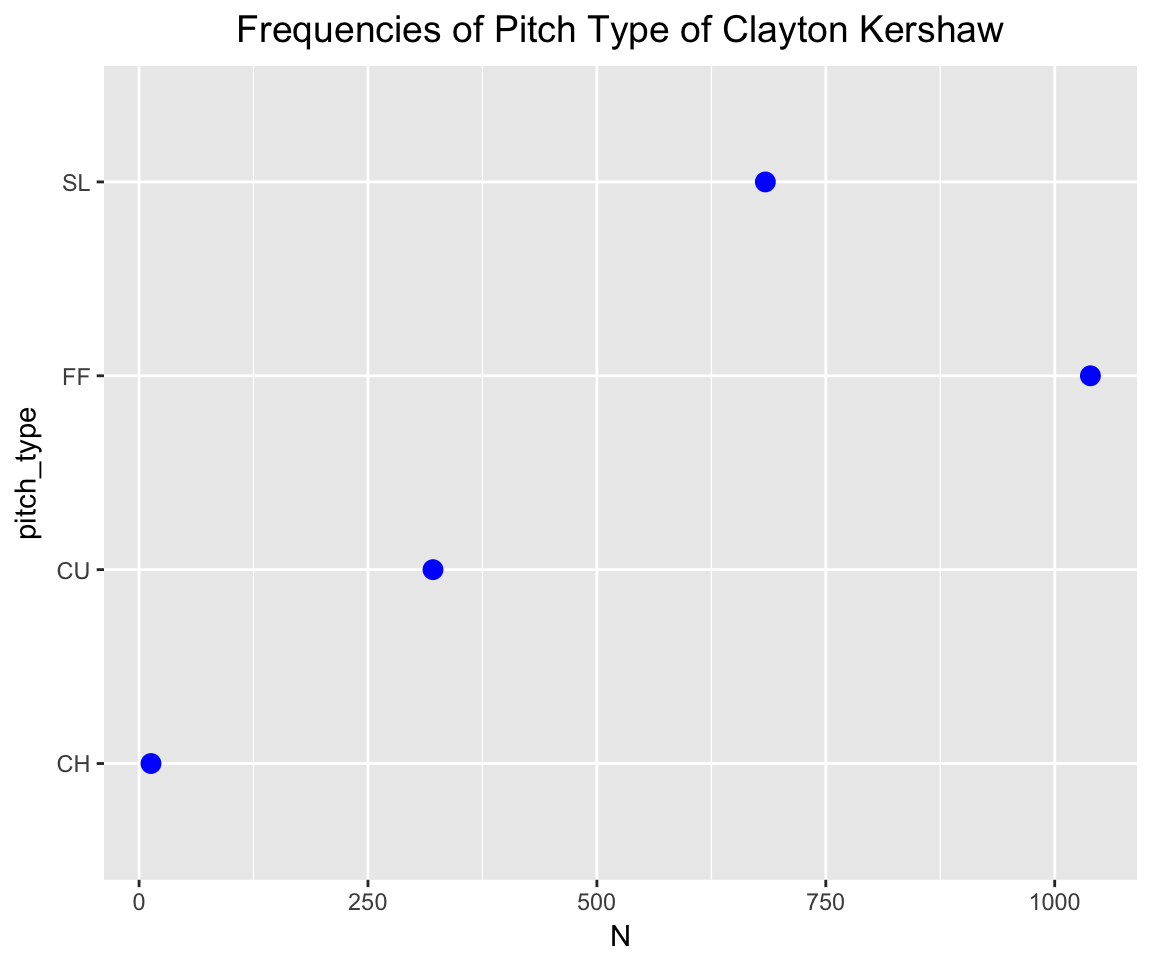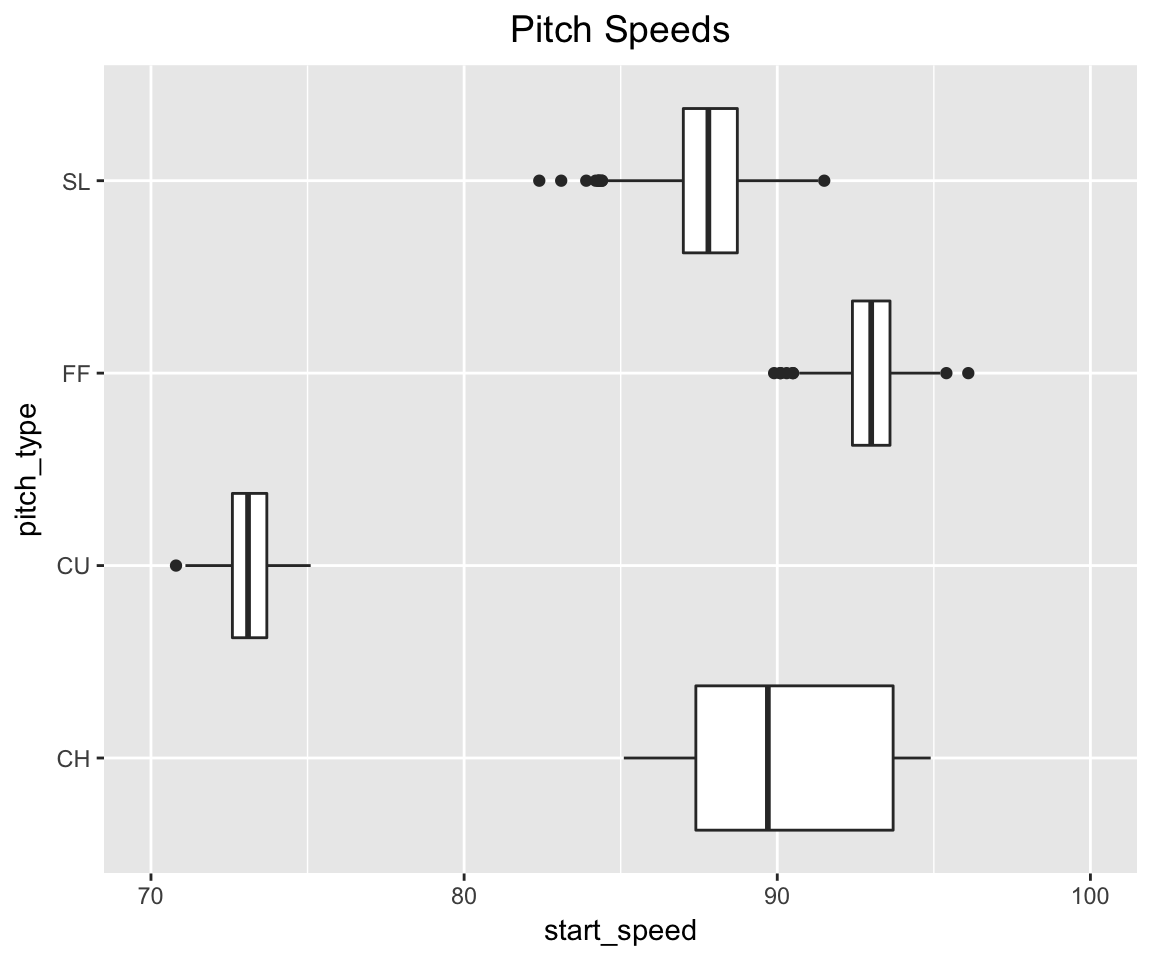``````library(dplyr)
library(ggplot2)
library(stringr)``````

#### The Data

Using the `pitchRX` package, I downloaded all of the pitch data for all games in the 2016 season. From this large dataset, I collected the data for 2044 pitches thrown by Clayton Kershaw.

Here I read in the pitchFX data and show a few lines.

``````library(readr)
``````## # A tibble: 6 x 15
##   pitch_type     px     pz             des   num
##        <chr>  <dbl>  <dbl>           <chr> <int>
## 1         FF  0.089  2.750   Called Strike     7
## 2         FF  0.083  2.721 Swinging Strike     7
## 3         FF -2.651  2.690            Ball     7
## 4         CU -0.644 -0.231            Ball     7
## 5         FF  0.642  4.521            Ball     7
## 6         FF -1.410  2.327 Swinging Strike     7
## # ... with 10 more variables: gameday_link <chr>, start_speed <dbl>,
## #   spin_dir <dbl>, spin_rate <dbl>, pfx_x <dbl>, pfx_z <dbl>, type <chr>,
## #   pitcher_name <chr>, event <chr>, stand <chr>``````

Here are the variables in the data frame `CK`.

• pitch_type - type of pitch thrown
• px - horizontal location in zone
• pz - vertical location in zone
• des - outcome of pitch
• start_speed - speed of pitch as it leaves the pitcher’s hand
• event - outcome of the plate appearance
• stand

``````library(ggplot2)
library(dplyr)``````

#### Pitch Types Thrown

To get an understanding of what pitch types are thrown, we construct a dotplot of the frequencies of the pitch types (variable `pitch_type`).

``````S_CK <- filter(summarize(group_by(CK, pitch_type),
N=n()),
pitch_type %in% c("SL", "FF", "CU", "CH"))
ggplot(S_CK, aes(pitch_type, N)) +
geom_point(size=3, color="blue") +
coord_flip() +
ggtitle("Frequencies of Pitch Type of Clayton Kershaw") +
theme(plot.title = element_text(size = 14,
hjust = 0.5))``````#### Pitch Speeds

These different pitch types are thrown at different speeds. The following display is a boxplot of the speeds (varialbe `start_speed`) of the four types of pitches thrown by Kershaw.

``````ggplot(filter(CK, pitch_type %in%
c("SL", "FF", "CU", "CH")),
aes(pitch_type, start_speed)) +
geom_boxplot() + coord_flip() +
ggtitle("Pitch Speeds") +
theme(plot.title = element_text(size = 14,
hjust = 0.5)) +
ylim(70, 100)``````#### Pitch Breaks

These pitch types are also distinguished by their movement or break. The variables `pfx_x` and `pfx_z` give the horizontal and vertical break amounts. (The perspective is from the catcher behind the plate.) The following graph shows the movements for each type of pitch.

``````CK <- filter(CK, pitch_type %in% c("CU",
"FF", "SL"))
ggplot(CK,
aes(pfx_x, pfx_z, shape=pitch_type)) +
geom_point(color="blue", size=2, alpha=0.5) +
ggtitle("Pitch Breaks") +
theme(plot.title = element_text(size = 14,
hjust = 0.5)) +
xlab("Horizontal Break") + ylab("Vertical Break")``````